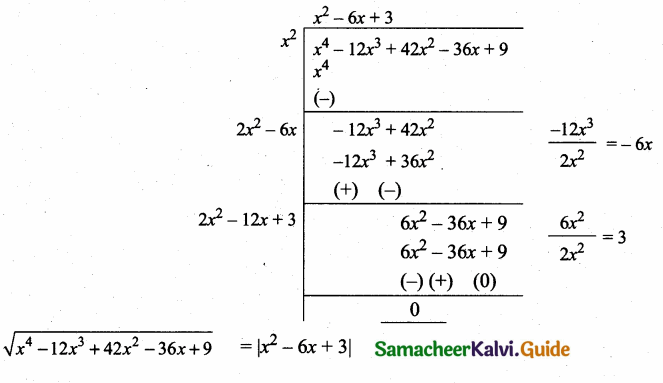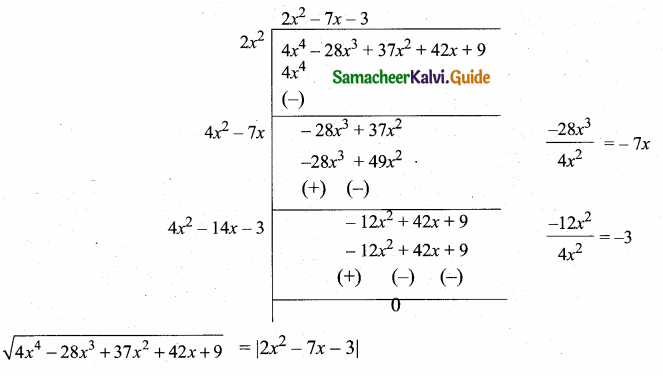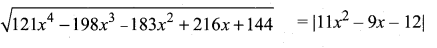Students can download Maths Chapter 3 Algebra Ex 3.8 Questions and Answers, Notes, Samacheer Kalvi 10th Maths Guide Pdf helps you to revise the complete Tamilnadu State Board New Syllabus, helps students complete homework assignments and to score high marks in board exams.

## Tamilnadu Samacheer Kalvi 10th Maths Solutions Chapter 3 Algebra Ex 3.8

Question 1.
Find the square root of the following polynomials by division method
(i) x4 – 12x3 + 42x2 – 36x + 9(ii) 31 x2 – 28x3 + 4x4 + 42x + 9
Rearrange the order we get
4x4 – 28x3 + 37x2 + 42x + 9(iii) 16x4 + 8x2 + 1(iv) 121 x4 – 198x3 – 183x2 + 216x + 144Question 2.
Find the square root of the expression
$$\frac{x^{2}}{y^{2}}$$ – $$\frac { 10x }{ y }$$ + 27 – $$\frac { 10y }{ x }$$ + $$\frac{y^{2}}{x^{2}}$$Question 3.
Find the values of a and b if the following polynomials are perfect squares.
(i) 4x4 – 12x3 + 37x2 + bx + aSince it is a perfect square
b + 42 = 0
b = – 42
a – 49 = 0
a = 49
∴ The value of a = 49 and b = – 42

(ii) ax4 + bx3 + 361x2 + 220x + 100
Re-arrange the order we get
100 + 220x + 361x2 + bx3 + ax4Since it is a perfect square
b – 264 = 0
b = 264
a – 144 = 0
a = 144
∴ The value of a = 144 and b = 264Question 4.
Find the values of m and n if the following expressions are perfect sqaures.
(i) $$\frac{1}{x^{4}}$$ – $$\frac{6}{x^{3}}$$ + $$\frac{13}{x^{2}}$$ + $$\frac { m }{ x }$$ + nSince it is a perfect square
$$\frac { 1 }{ x }$$ (m + 12) = 0
m + 12 = 0
m = -12
n – 4 = 0
n = 4
∴ The value of m = -12 and n = 4(ii) x4 – 8x3 + mx2 + nx + 16